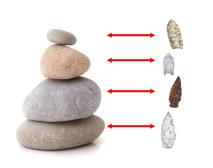## Cardinality and Infinite Sets

In the proof of the Chinese Remainder Theorem, a key step was showing that two sets must have the same number of elements if we can find a way to "pair up" every element from one set with one and only one element from the other, and vice-versa. Notice, this idea gives us the ability to compare the "sizes" of sets without ever counting their elements.

For example, Grog the Caveman can tell -- without ever counting to four -- that the pile of rocks and the bunch of arrow-heads shown below are of the same "size" by pairing them up in the manner shown below, and noting that there was nothing left over from either group:Now think about sets of things that we have difficulty counting...
• How many integers are there?

• How many fractions exist? ... or real numbers?

• How many points lie on a plane?

One might say "infinitely many" in each case above -- but what does that really mean? More interestingly, can one of these infinite sets be fundamentally "bigger" than another in some way, or is every infinite set the "same size" as any other infinite set?

Let us adopt the mindset of Grog the Caveman. Let us say that two sets have the same "size" if a "pairing" between their elements exists.

We really need to be more precise here, as otherwise, the ambiguities of the English language might cause us problems.

To this end, let us adopt the following notation...

When we wish to discuss a function $f(x)$ which takes as inputs elements from a set $A$ (the domain of $f$) and outputs elements contained in some specified set $B$ (referred to as the co-domain for $f$), we write $$f : A \rightarrow B$$

Let us also make the following definitions:

$f : A \rightarrow B$ is injective if the only time $f(a_1)=f(a_2)$ happens is when $a_1=a_2$.

$f : A \rightarrow B$ is surjective (or onto) if given any element $b$ in set $B$, we can always find some element $a$ in $A$, so that $f(a)=b$.

$f : A \rightarrow B$ is a called a bijection (or one-to-one correspondence) if it is both injective and surjective.

The "pairings" we were talking about before can now be more precisely described as bijections.

Similarly, we define the cardinality of a set $A$, denoted $|A|$, as a more generalized version of the "size" of a set that allows for the discussion of sets with an infinite number of elements. Specifically, we define it in such a way so that:

1. Two sets $A$ and $B$ have the same cardinality ("size") if there exists a bijection ("pairing") between them.
2. Set $A$ has smaller cardinality than set $B$ if there is an injective function, but no bijective function, from $A$ to $B$.

Now, for the strange results that arise as a consequence of these definitions...

We can see that the set of natural numbers, $\{1,2,3,4...\}$, and the set of positive even numbers, $\{2,4,6,8...\}$, have the same cardinality (i.e., "size") as the bijective function $f(x)=2x$ takes elements from the former to the latter.

Similarly, we can see the the set of natural numbers, $\{1,2,3,4...\}$, have the same cardinality (i.e., "size") as the set of integers, $\{...,-4,-3,-2,-1,0,1,2,3,4...\}$, by finding the bijection shown below:
$$\begin{array}{c|c} x & f(x)\\\hline 1 & 0\\ 2 & 1\\ 3 & -1\\ 4 & 2\\ 5 & -2\\ 6 & 3\\ 7 & -3\\ \vdots & \vdots\\ \end{array}$$

Indeed, this last comparison reveals that any set that is "listable" (i.e., all of its elements can be written in a list) must have the same cardinality as the natural numbers. We call such sets countable. To make the argument that the cardinalities agree, simply define a function $f(x)$ where $f(1)$ is the first element in the list, $f(2)$ is the second element, $f(3)$ is the third, etc... This technique lies at the heart of Cantor's arguments that show the set of all rationals (i.e., fractions) also agrees with the cardinality of the natural numbers, while the set of reals are in some strange way, somehow fundamentally "bigger".